﻿ 基于多产品报童模型的自动售货机补货策略研究

# 基于多产品报童模型的自动售货机补货策略研究Replenishment Strategy of Vending Machine Based on Multi Product Newsboy Model

Abstract: This paper takes the vending machine of a brand in Shanghai Metro as the research object. Consid-ering that vending machine is different from other traditional stores, each commodity has its own fixed aisle and its capacity is limited. Moreover, frequent out of stock will bring poor consumption experience to customers, so the service will be provided. Considering the level and taking the de-mand distribution forecast as the input, a multi product newsboy replenishment model based on the dual constraints of channel capacity and service level is constructed. Lagrange function and KKT multiplier are introduced to solve the model. The results show that: 1) Compared with the original way of replenishing all the vending machines in each replenishment period, the multi product newsboy replenishment model based on the dual constraints of channel capacity and service level can bring more benefits. 2) Considering the service level constraint, with the increase of service lev-el, the cost increases faster and faster, so the operating enterprises need to choose a reasonable service level to control the cost at a lower level.

1. 引言

2. 文献综述

3. 模型建立

3.1. 问题描述

3.2. 创建模型

${p}_{i}$ ：产品i的售价；

${c}_{i}$ ：产品i的成本；

${X}_{i}$ ：产品i的需求；

${\mu }_{i}$${X}_{i}$ 的均值；

${\sigma }_{i}$${X}_{i}$ 的方差；

${f}_{i}\left(x\right)$${X}_{i}$ 的概率密度函数；

${F}_{i}\left(x\right)$${X}_{i}$ 的累积分布函数；

${Q}_{i}$ ：产品i补货后最优量；

s：每件产品的运输费用；

G：每次补货的固定成本；

${R}_{i}$ ：产品i的货道容量；

${L}_{i}$ ：售货机产品i剩余量。

1) 产品之间需求相互独立。

2) 不失一般性， ${p}_{i}-{c}_{i}>0$${p}_{i}-{c}_{i}-s>0$

3) 根据文献所提出的衡量标准，服务水平的定义为

$ES{L}_{i}=1-\frac{E{S}_{i}}{{\mu }_{i}}$ (1)

$ESL={\sum }_{i=1}^{n}{w}_{i}\ast ES{L}_{i}$ (2)

4) ${F}_{i}\left(x\right)$ 连续可微，且当 $x>0$ 时， ${F}_{i}\left(x\right)$ 是严格单调的函数。

$E\left(Q\right)=\underset{i=1}{\overset{n}{\sum }}\left(\left({p}_{i}-{c}_{i}\right){\int }_{0}^{{Q}_{i}}x{f}_{i}\left(x\right)+\left({p}_{i}-{c}_{i}\right){\int }_{{Q}_{i}}^{\infty }{Q}_{i}{f}_{i}\left(x\right)\text{d}x-s\left({Q}_{i}-{L}_{i}\right)\right)-G$ (3)

$\mathrm{max}E\left(Q\right)$ (4)

$\text{s}\text{.t}\text{\hspace{0.17em}}\text{\hspace{0.17em}}0\le {Q}_{i}\le {R}_{i},i=1,2,\cdots ,n$ (5)

${\sum }_{i=1}^{n}\frac{{w}_{i}}{{\mu }_{i}}{\int }_{{Q}_{i}}^{\infty }\left(x-{Q}_{i}\right){f}_{i}\left(x\right)\text{d}x\le \alpha$ (6)

3.3. 模型分析与算法

$L=-E\left(Q\right)+\underset{i=1}{\overset{n}{\sum }}{\beta }_{i}\left({Q}_{i}-{R}_{i}\right)+\lambda \left(\underset{i=1}{\overset{n}{\sum }}\frac{{w}_{i}}{{\mu }_{i}}{\int }_{{Q}_{i}}^{\infty }\left(x-{Q}_{i}\right){f}_{i}\left(x\right)\text{d}x-\alpha \right)$ (7)

${Q}_{i}^{\ast }={F}_{i}^{-1}\left(\frac{{p}_{i}-{c}_{i}-s}{{p}_{i}-{c}_{i}}\right),i=1,2,\cdots ,n$ (8)

$\frac{\partial L}{\partial {Q}_{i}}=\left({p}_{i}-{c}_{i}+\lambda \frac{{w}_{i}}{{\mu }_{i}}\right){F}_{i}\left({Q}_{i}\right)-\left({p}_{i}-{c}_{i}-s-{\beta }_{i}+\lambda \frac{{w}_{i}}{{\mu }_{i}}\right)=0$ (9)

${\beta }_{i}\left({Q}_{i}-{R}_{i}\right)=0$ (10)

$\lambda \left({\sum }_{i=1}^{n}\frac{{w}_{i}}{{\mu }_{i}}{\int }_{{Q}_{i}}^{\infty }\left(x-{Q}_{i}\right){f}_{i}\left(x\right)\text{d}x-\alpha \right)=0$ (11)

$\lambda \le \frac{{\mu }_{i}}{{w}_{i}}\left({c}_{i}-{p}_{i}+\frac{s}{1-F\left({R}_{i}\right)}\right)$ (12)

${\Delta }_{i}=\frac{{\mu }_{i}}{{w}_{i}}\left({c}_{i}-{p}_{i}+\frac{s}{1+F\left({R}_{i}\right)}\right)$$\left(i=1,2,\cdots ,n\right)$，假设 ${\Delta }_{i}$ 满足 ${\Delta }_{1}\le {\Delta }_{2}\le \cdots \le {\Delta }_{n}$。当 $0\le \lambda \le {\Delta }_{i}$ 时，方程(9)和(10)的解满足

${\beta }_{i}=0$, ${F}_{i}\left({Q}_{i}\right)=\frac{{p}_{i}-{c}_{i}-s+\lambda \frac{{w}_{i}}{{\mu }_{i}}}{{p}_{i}-{c}_{i}+\lambda \frac{{w}_{i}}{{\mu }_{i}}}$ (13)

$\lambda >{\Delta }_{i}$ 时， ${\beta }_{i}>0$，方程2.10的解满足 ${Q}_{i}={R}_{i}$。下面证明存在 ${Q}_{i}={R}_{i}$ 对于方程(9)成立。

${\beta }_{i}>0$ 时，此时 ${Q}_{i}>{R}_{i}$，根据 ${F}_{i}\left(·\right)$ 定义以及严格单调性可知， ${F}_{i}^{\ast }\left({Q}_{i}\right)>F\left({R}_{i}\right)$。由方程(13)得到

${F}_{i}^{\ast }\left({Q}_{i}\right)=\frac{{p}_{i}-{c}_{i}-s+\lambda \frac{{w}_{i}}{{\mu }_{i}}}{{p}_{i}-{c}_{i}+\lambda \frac{{w}_{i}}{{\mu }_{i}}}>F\left({R}_{i}\right),\text{\hspace{0.17em}}\text{\hspace{0.17em}}{F}_{i}\left({Q}_{i}\right)=\frac{{p}_{i}-{c}_{i}-s-{\beta }_{i}+\lambda \frac{{w}_{i}}{{\mu }_{i}}}{{p}_{i}-{c}_{i}+\lambda \frac{{w}_{i}}{{\mu }_{i}}}=F\left({R}_{i}\right)$ (14)

${\beta }_{i}=\left(1-F\left({R}_{i}\right)\right)\left({p}_{i}-{c}_{i}+\lambda \frac{{w}_{i}}{{\mu }_{i}}\right)-s,\text{\hspace{0.17em}}{Q}_{i}={R}_{i}>0$(15)

$G\left(\lambda \right)={\sum }_{i=1}^{n}\frac{{w}_{i}}{{\mu }_{i}}{\int }_{{Q}_{i}}^{\infty }\left(x-{Q}_{i}\right){f}_{i}\left(x\right)\text{d}x-\alpha ,\left(i=1,2,\cdots ,n\right)$ (16)

$\frac{\partial G\left(\lambda \right)}{\partial {Q}_{i}\left(\lambda \right)}=-\frac{{w}_{i}}{{\mu }_{i}}\left(1-{F}_{i}\left({Q}_{i}\right)\right)$ (17)

${f}_{i}\left({Q}_{i}\right)\cdot \frac{\text{d}{Q}_{i}}{\text{d}\lambda }=\frac{{w}_{i}}{{\mu }_{i}}\cdot \frac{s+\beta }{{\left(-{c}_{i}+{p}_{i}+\frac{\lambda {w}_{i}}{{\mu }_{i}}\right)}^{2}{\mu }_{i}}$ (18)

$0\le \lambda \le {\Delta }_{1}$ 时，根据定理1可以得到，对于任意 $i=1,2,\cdots ,n$

${\beta }_{i}=0$, ${F}_{i}\left({Q}_{i}\right)=\frac{{p}_{i}-{c}_{i}-s+\lambda \frac{{w}_{i}}{{\mu }_{i}}}{{p}_{i}-{c}_{i}+\lambda \frac{{w}_{i}}{{\mu }_{i}}}$ (19)

$G\left(\lambda \right)={\sum }_{i=1}^{n}\frac{{w}_{i}}{{\mu }_{i}}{\int }_{{Q}_{i}}^{\infty }\left(x-{Q}_{i}\right){f}_{i}\left(x\right)\text{d}x-\alpha ,\left(i=1,2,\cdots ,n\right)$ (20)

$\lambda >{\Delta }_{1}$ 时，假设 ${\Delta }_{k}\le \lambda <{\Delta }_{k+1}$$k=1,2,\cdots ,n$，则对于任意 $i=1,2,\cdots ,k$

${\beta }_{i}=\left(1-F\left({R}_{i}\right)\right)\left({p}_{i}-{c}_{i}+\lambda \frac{{w}_{i}}{{\mu }_{i}}\right)-s,\text{\hspace{0.17em}}{Q}_{i}={R}_{i}$ (21)

$G\left(\lambda \right)=\underset{i=1}{\overset{k}{\sum }}\frac{{w}_{i}}{{\mu }_{i}}{\int }_{{R}_{i}}^{\infty }\left(x-{R}_{i}\right){f}_{i}\left(x\right)\text{d}x+\underset{i=k+1}{\overset{n}{\sum }}\frac{{w}_{i}}{{\mu }_{i}}{\int }_{{Q}_{i}}^{\infty }\left(x-{R}_{i}\right){f}_{i}\left(x\right)\text{d}x-\alpha$ (22)

${\sum }_{i=1}^{n}\frac{{w}_{i}}{{\mu }_{i}}{\int }_{{R}_{i}}^{\infty }\left(x-{R}_{i}\right){f}_{i}\left(x\right)\text{d}x-\alpha <0$ (23)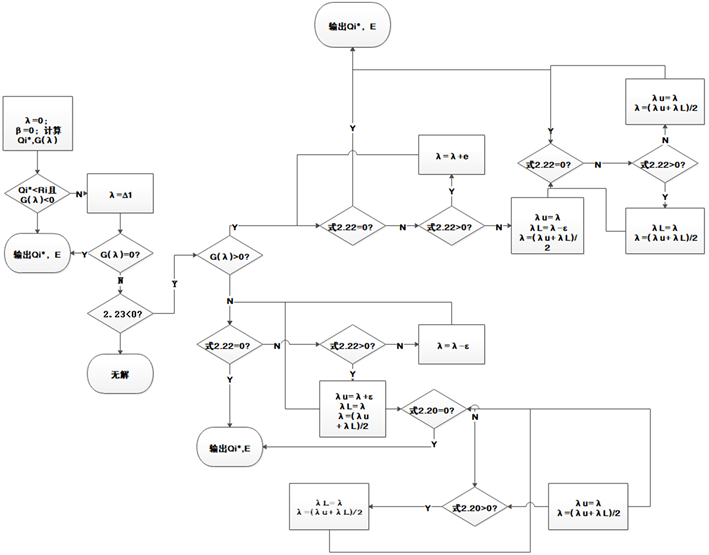Figure 1. Algorithm flow chart of solving the optimal replenishment quantity

Step 0 输入参数， $\epsilon >0$ ；取值 $\lambda =0$$\beta =0$，根据式2.8计算 ${Q}_{i}^{\ast }$，若 ${Q}_{i}^{\ast }\le {R}_{i}$$G\left(\lambda \right)<0$，转step 15。

Step 1 计算 ${\Delta }_{i}$，令 ${\Delta }_{1}\le {\Delta }_{2}\le \cdots \le {\Delta }_{n}$$\lambda$ 取值 ${\Delta }_{1}$，代入式2.16计算 $G\left(\lambda \right)$。如果 $G\left(\lambda \right)=0$，转step 15；如果 $G\left(\lambda \right)>0$，转step 2；如果 $G\left(\lambda \right)<0$，转step 9。

Step 2 根据式(23)进行计算，如果式(23)值不成立，输出“无解”；否则转step 3。

Step 3 计算满足下面条件的整数k： ${\Delta }_{1}\le \cdots \le {\Delta }_{k}\le \lambda \le {\Delta }_{k+1}\le \cdots \le {\Delta }_{n}$，代入式2.22计算 $G\left(\lambda \right)$。若 $G\left(\lambda \right)=0$，转step 15；若 $G\left(\lambda \right)>0$，转step 4；若 $G\left(\lambda \right)<0$，转step 5。

Step 4 $\lambda =\lambda +\epsilon$，转step 3。

Step 5 令 ${\lambda }_{U}=\lambda +\epsilon$${\lambda }_{L}=\lambda$$\lambda =\frac{{\lambda }_{U}+{\lambda }_{L}}{2}$

Step 6 将 代入式2.22计算 $G\left(\lambda \right)$。若 $G\left(\lambda \right)=0$，转step 15；若 $G\left(\lambda \right)>0$，转step 7；若 $G\left(\lambda \right)<0$，转step 8。

Step 7 令 ${\lambda }_{L}=\lambda$$\lambda =\frac{{\lambda }_{U}+{\lambda }_{L}}{2}$，转step 6。

Step 8 令 ${\lambda }_{U}=\lambda$$\lambda =\frac{{\lambda }_{U}+{\lambda }_{L}}{2}$，转step 6。

Step 9 令 $\lambda =\lambda -\epsilon$，转step 10。

Step 10 根据2.20计算 $G\left(\lambda \right)$，若 $G\left(\lambda \right)=0$，转step 15；若 $G\left(\lambda \right)<0$，转step 11；若 $G\left(\lambda \right)>0$，转step 5。

Step 11 令 $\lambda =\lambda -\epsilon$，转step 10。

Step 12 将 $\lambda$ 代入2.20计算 $G\left(\lambda \right)$，若 $G\left(\lambda \right)=0$，转step 15；若 $G\left(\lambda \right)<0$，转step 13；若 $G\left(\lambda \right)>0$，转step 14。

Step 13 令 ${\lambda }_{U}=\lambda$$\lambda =\frac{{\lambda }_{U}+{\lambda }_{L}}{2}$，转step 12。

Step 14 令 ${\lambda }_{L}=\lambda$$\lambda =\frac{{\lambda }_{U}+{\lambda }_{L}}{2}$，转step 12。

Step 15 代入式2.5，计算 $E\left({Q}_{i}\right)$，输出 ${Q}_{i}^{\ast }$

4. 实验仿真

4.1. 数据准备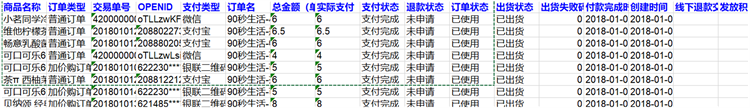Figure 2. Example of sales flow of a vending machineTable 1. Statistical example of daily sales volume of each commodity of a single vending machineTable 2. Description of sales characteristics of a certain cargo aircraft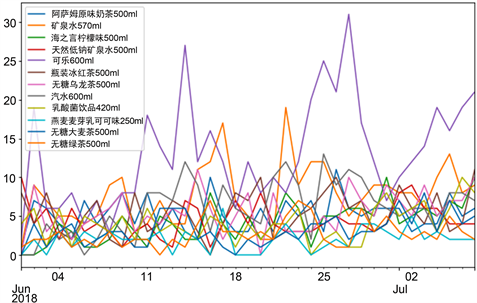Figure 3. Sales statistics time series chart of a certain vending machine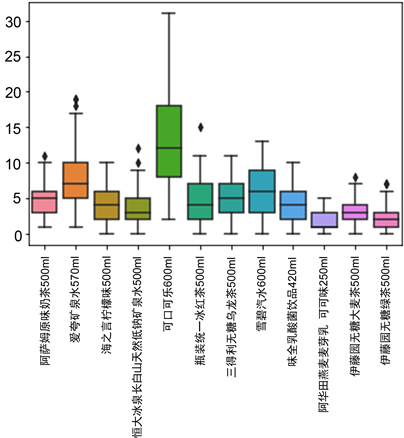Figure 4. Box diagram of sales volume of a certain freighter

4.2. 基于货道容量和服务水平双重约束的多产品报童补货模型实证

${w}_{i}={p}_{i}{u}_{i}/\underset{i=1}{\overset{n}{\sum }}{p}_{i}{u}_{i}$Table 4. Optimal inventory of goodsTable 5. The relationship between optimal inventory and S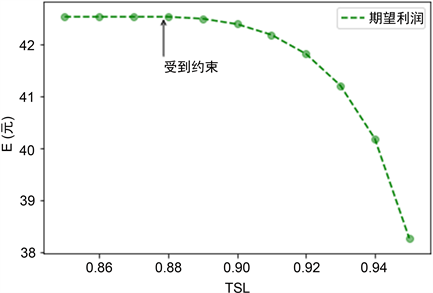Figure 5. Schematic diagram of the relationship between optimal profit and service levelTable 6. The change of optimal inventory with S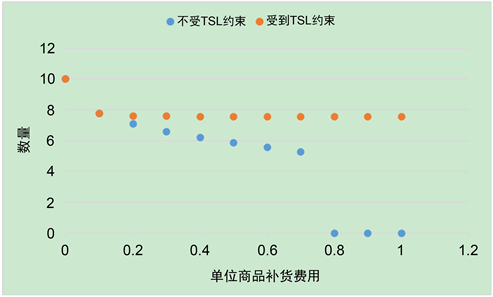Figure 6. The optimal inventory under TSL constraint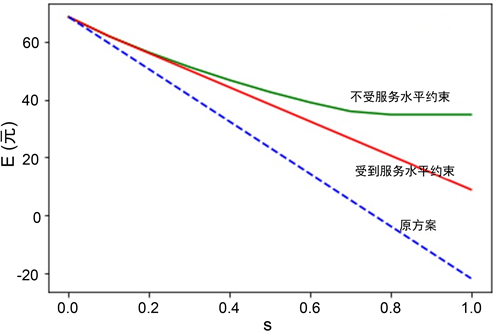Figure 7. The relationship between model profit and S

5. 结论

 Moon, I. and Silver, E.A. (2000) The Multi-Item Newsvendor Problem with a Budget Constraint and Fixed Ordering Costs. Journal of the Operational Research Society, 51, 602-608.
https://doi.org/10.1057/palgrave.jors.2600938

 Abdel-Malek, L.L. and Montanari, R. (2005) On the Mul-ti-Product Newsboy Problem with Two Constraints. Computers & Operations Research, 32, 2095-2116.
https://doi.org/10.1016/j.cor.2004.02.002

 Abdel-Malek, L.L. and Areeratchakul, N. (2007) A Quadratic Pro-gramming Approach to the Multi-Product Newsvendor Problem with Side Constraints. European Journal of Operational Research, 176, 1607-1619.
https://doi.org/10.1016/j.ejor.2005.11.002

 Niederhoff, J.A. (2007) Using Separable Programming to Solve the Multi-Product Multiple Ex-Ante Constraint Newsvendor Problem and Extensions. European Journal of Operational Research, 176, 941-955.
https://doi.org/10.1016/j.ejor.2005.09.046

 Erlebacher, S.J. (2000) Optimal and Heuristic Solutions for the Multi-Item Newsvendor Problem with a Single Capacity Constraint. Production and Operations Management, 9, 303-318

 Zhang, G. (2010) The Multi-Product Newsboy Problem with Supplier Quantity Discounts and a Bud­Get Constraint. European Journal of Operational Research, 206, 350-360.
https://doi.org/10.1016/j.ejor.2010.02.038

Top